Courses
Courses for Kids
Free study material
Free LIVE classes
More

# CBSE Class 7 Maths The Triangle and Its Properties Notes - Chapter 6 Free PDFLIVE
Join Vedantu’s FREE Mastercalss

## Revision Notes for CBSE Class 7 Maths Chapter 6 - Free PDF Download

The Triangle and its properties included under CBSE Class 7 Maths syllabus is an important chapter to know about the various characteristics of triangles. Therefore, one must go through these notes that are prepared by our Maths expert. Class 7 Maths The Triangle and Its Properties Revision Notes provided on this page can be downloaded in free PDF format and one can easily refer to these notes to brush up on all the important concepts related to this chapter.

Revision Notes for CBSE Class 7 Maths Chapter 6  are prepared to help students during the last-minute revision. The keynotes that are provided in these PDFs include all the topics and information that are covered in the chapter.

## Class 7 Maths The Triangle and Its Properties - Important Topics

The important topics that are covered in CBSE Class 7 Maths Chapter 6 The Triangle and Its Properties are mentioned below:

• Triangles and their basic elements.

• Types of triangles based on their sides (Equilateral, Isosceles and Scalene)

• Types of triangles based on their angle measurement (Right-angled, obtuse-angled and acute-angled).

• Properties of a triangle.

Also, check CBSE Class 7 Maths revision notes for All chapters:

Last updated date: 23rd Sep 2023
Total views: 572.1k
Views today: 7.72k

## Access Class 7 Mathematics Chapter 6 - The Triangle and its Properties Notes

Triangles: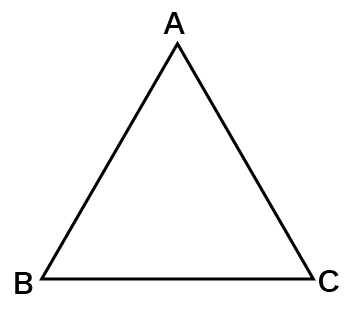• Triangles are three line segments that form a closed plane figure.

• In above figure, $\vartriangle \text{ABC}$ is a triangle.

• The three angles and three sides of a triangle make up the triangle's six constituents.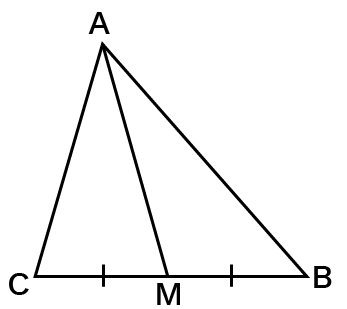• The line segment connecting a triangle's vertex to the opposite side's midpoint is known as the triangle's median.

• In above figure, $\text{AM}$ is the median of triangle $\text{ABC}$.

• There are three medians in a triangle.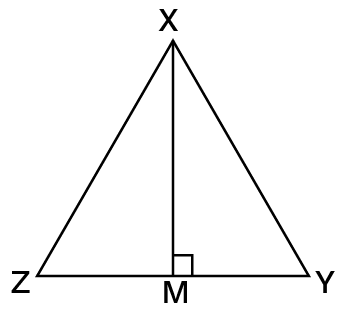• The perpendicular line segment connecting a triangle's vertex to its opposite side is known as the triangle's height or altitude of a triangle.

• In above figure, $\text{XM}$ is the altitude (height) of triangle $\text{XYZ}$.

• There are three elevations in a triangle.

Type of Triangle Based on Sides:

• Equilateral Triangle: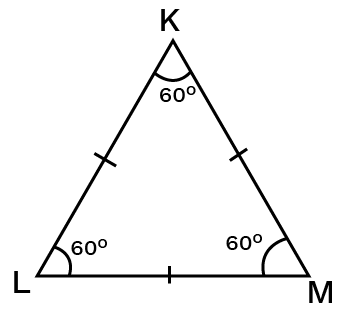If each of a triangle's sides is the same length, then the triangle is said to be equilateral.

Each angle in an equilateral triangle is $60$ degrees.

• Isosceles Triangle: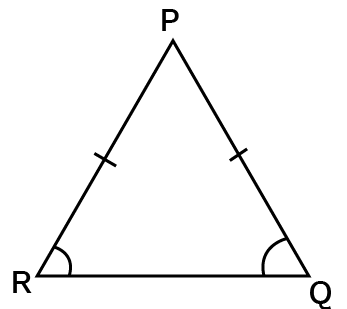If at least two of the triangle's sides are the same length, then it is said to be isosceles.

• An isosceles triangle's base is its non-equal side.

• The base angles of an isosceles triangle have equal measures.

• Scalene Triangle: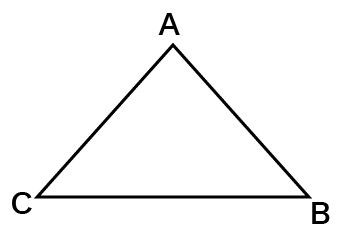• All of the sides of a triangle are different lengths.

• There are no two angles that are the same.

Property of the Lengths of Sides of a Triangle:

• Any two sides of a triangle have a length that is more than the length of the third side.

• The length difference between any two sides is smaller than the length difference between the third and fourth sides.

• This feature is important for determining whether or not a triangle may be drawn when the lengths of the three sides are known.

Types of Triangle-based on Angles:

1. Right Angled Triangle: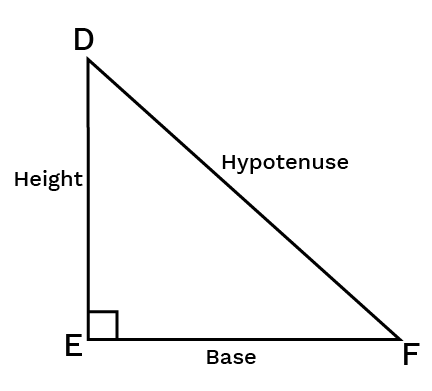• A triangle in which one of the angles is measured in ${{90}^{0}}$.

• The hypotenuse is the side opposite the right angle of a right-angled triangle, whereas the legs are the other two sides.

1. Obtuse Angled Triangle: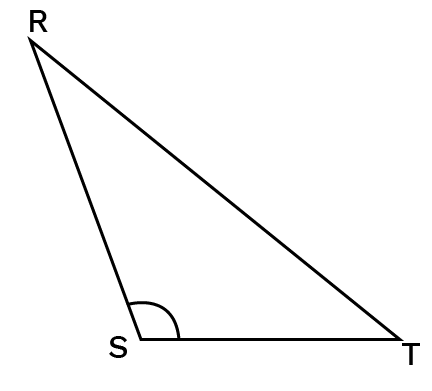A triangle in which one of the angles is larger than ${{90}^{0}}$.

1. Acute Angled Triangle: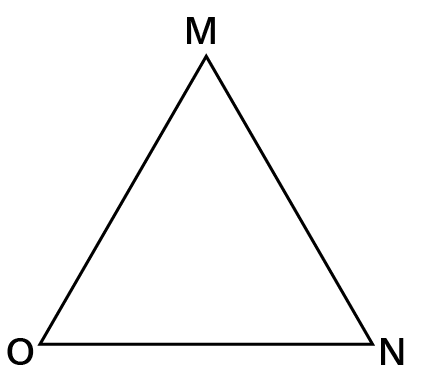A triangle in which each of the angles is smaller than ${{90}^{0}}$.

Pythagoras Property: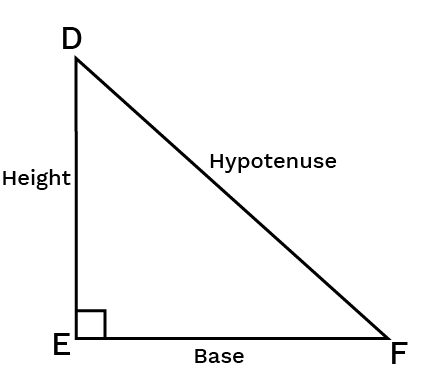• In right-angled triangle, the square on the hypotenuse equals the sum of the squares on the legs of a right-angled triangle.

• Triangle $\text{DEF}$ is a right angled triangle. According to Pythagoras Theorem,

${{\left( Hypotenuse \right)}^{2}}\text{ }=\text{ S}um\text{ }of\text{ }the\text{ }squares\text{ }on\text{ }the\text{ }legs.$

Therefore,

${{\left( \text{DF} \right)}^{2}}={{\left( \text{DE} \right)}^{2}}+{{\left( \text{EF} \right)}^{2}}$

• This property does not hold true if a triangle is not right-angled.

• This feature can be used to determine whether or not a triangle is right-angled.

Exterior Angle: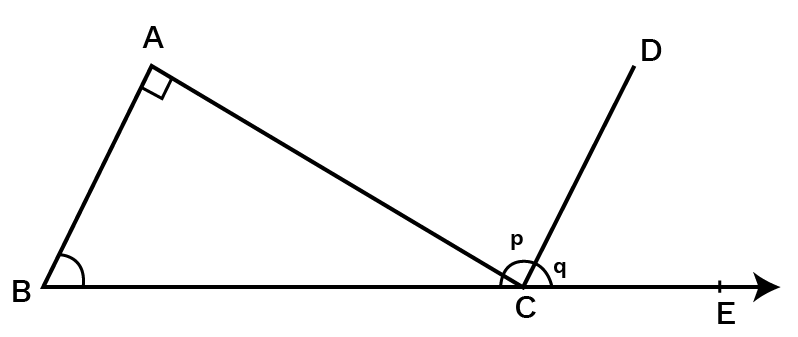• The sum of a triangle's internal opposite angles equals its outer angle that is known as the Exterior angle of a triangle.

• In above figure, $\angle \text{ACE}$ is an Exterior angle.

$\angle \text{ACE = }\angle \text{A}+\angle \text{B}$

• When a side of a triangle is made, an external angle of a triangle is formed.

• There are two ways to generate an external angle at each vertex.

A Property of Exterior Angles:

The sum of the measures of a triangle's internal opposite angles equals the measure of any of its outer angles.

The Angle Sum Property of a Triangle:

The total measure of a triangle's three angles is ${{180}^{0}}$.

## Property of the Lengths of Sides of a Triangle:

• The lengths of any two sides of a triangle are always bigger than the third side's length.

• The length difference between any two triangle sides is always less than the length of the third side.

## Benefits of CBSE Class 7 Maths Chapter 6 Revision Notes

Here are a few points that tell us the benefits of CBSE Class 7 Maths Revision Notes:

• These notes are your one-stop solution for last-minute exam preparation.

• The topic-wise key points included in these revision notes will help you to revise all the chapters easily and also to brush up on the concepts.

• Class 7 Maths Chapter 6 Revision Notes are made by the subject experts at Vedantu, so you can rely on these answers completely.

• Referring to the CBSE Class 7 Maths Chapter 6 Revision Notes will help students improve their understanding of all the topics which are provided here in a detailed manner.

## Conclusion

Therefore, Class 7 Maths Revision Notes Chapter 6 is not only helpful for your exam preparation but will also aid you in getting a deeper understanding of all the topics. Download the free PDF today and start your preparation with these notes to score excellent marks. You can also refer to NCERT Solutions for Class 7 Maths to revise the complete syllabus and score more marks in your examinations.

## FAQs on CBSE Class 7 Maths The Triangle and Its Properties Notes - Chapter 6 Free PDF

1. What is a Pythagoras Theorem?

The Pythagoras Theorem is an important concept in Mathematics which explains the property of a right-angled triangle. The theorem states that the sum of the individual squares of the base and the perpendicular of a right-angled triangle is equal to the square of the hypotenuse.

2. What is an obtuse triangle?

An obtuse triangle can be defined as the triangle whose one angle is greater than 90 degrees and the sum of all the three interior angles equals 180 degrees.

3. What is an equilateral triangle?

The triangle where all the sides are equal in length and all the three interior angles measure 60 degrees each, then the triangle is known as an equilateral triangle.

4. What are the four properties of a triangle?

The four important properties of a triangle are:

• All the three angles of a triangle are always equal to 180 degrees.

• The sum of the length of two sides of any triangle is always greater than the length of the third side.

• The difference in lengths of any two sides of a triangle is always less than the length of the third side.

• The sum of the opposite interior angles of a triangle is equal to any of the exterior angles of the triangle.

5. Can all three sides of a triangle be different in length?

Yes, all three sides of a triangle can be of different lengths. Such triangles are known as scalene triangles. Here, the measure of all the three angles is different from each other but sums up to 180 degrees.